# Cartan subalgebra

(diff) ← Older revision | Latest revision (diff) | Newer revision → (diff)

of a finite-dimensional Lie algebraover a fieldA nilpotent subalgebra ofwhich is equal to its normalizer in. For example, ifis the Lie algebra of all complex square matrices of a fixed order, then the subalgebra of all diagonal matrices is a Cartan subalgebra in. A Cartan subalgebra can also be defined as a nilpotent subalgebrainwhich is equal to its Fitting null-component (cf. Weight of a representation of a Lie algebra)wheredenotes the adjoint representation (cf. Lie algebra) of.

Suppose further thatis of characteristic zero. Then for any regular element, the set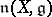of all elements ofwhich are annihilated by powers of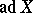is a Cartan subalgebra of, and every Cartan subalgebra ofhas the formfor some suitable regular element. Each regular element belongs to one and only one Cartan subalgebra. The dimension of all the Cartan subalgebras ofare the same and are equal to the rank of. The image of a Cartan subalgebra under a surjective homomorphism of Lie algebras is a Cartan subalgebra. Ifis algebraically closed, then all Cartan subalgebras ofare conjugate; more precisely, they can be transformed into another by operators of the algebraic groupof automorphisms ofwhose Lie algebra is the commutator subalgebra of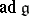. Ifis solvable, then the above assertion holds without the hypothesis thatbe algebraically closed.

Letbe either a connected linear algebraic group over an algebraically closed fieldof characteristic zero, or a connected Lie group, and letbe its Lie algebra. Then a subalgebraofis a Cartan subalgebra if and only if it is the Lie algebra of a Cartan subgroup of.

Letbe a subalgebra of the Lie algebra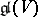of all endomorphisms of a finite-dimensional vector spaceover, and let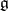be the smallest algebraic Lie algebra incontaining(cf. Lie algebra, algebraic). If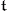is a Cartan subalgebra of, thenis a Cartan subalgebra of, and ifis a Cartan subalgebra ofandis the smallest algebraic subalgebra ofcontaining, thenis a Cartan subalgebra ofand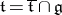.

Let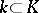be a field extension. A subalgebraofis a Cartan subalgebra if and only ifis a Cartan subalgebra of.

Cartan subalgebras play an especially important role whenis a semi-simple Lie algebra (this was used by E. Cartan ). In this case, every Cartan subalgebraofis Abelian and consists of semi-simple elements (see Jordan decomposition), and the restriction of the Killing form tois non-singular.

How to Cite This Entry:
Cartan subalgebra. Encyclopedia of Mathematics. URL: http://encyclopediaofmath.org/index.php?title=Cartan_subalgebra&oldid=12475
This article was adapted from an original article by V.L. Popov (originator), which appeared in Encyclopedia of Mathematics - ISBN 1402006098. See original article